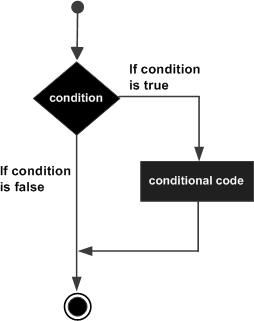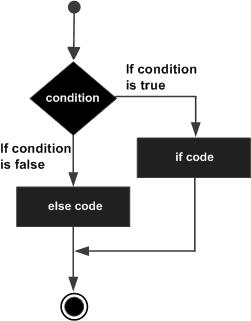Friday, 15 September 2017 07:42

## C-Decision Statements

Written by
Rate this item

Decision making structures require that the programmer specifies one or more conditions to be evaluated or tested by the program, along with a statement or statements to be executed if the condition is determined to be true, and optionally, other statements to be executed if the condition is determined to be false.

Show below is the general form of a typical decision making structure found in most of the programming languages −C programming language assumes any non-zero and non-null values as true, and if it is either zero or null, then it is assumed as false value.

C programming language provides the following types of decision making statements.

Statement & Description

### if statement

 An if statement consists of a boolean expression followed by one or more statements.

## Syntax

The syntax of an 'if' statement in C programming language is −

```if(boolean_expression) {
/* statement(s) will execute if the boolean expression is true */
}```

If the Boolean expression evaluates to true, then the block of code inside the 'if' statement will be executed. If the Boolean expression evaluates to false, then the first set of code after the end of the 'if' statement (after the closing curly brace) will be executed.

C programming language assumes any non-zero and non-null values as true and if it is either zero or null, then it is assumed as false value.

## Flow Diagram

``
```#include <stdio.h>

int main () {

/* local variable definition */
int a = 10;

/* check the boolean condition using if statement */

if( a < 20 ) {
/* if condition is true then print the following */
printf("a is less than 20\n" );
}

printf("value of a is : %d\n", a);

return 0;
}When the above code is compiled and executed, it produces the following result −```
```a is less than 20;
value of a is : 10```

### if...else statement

An if statement can be followed by an optional else statement, which executes when the Boolean expression is false.

An if statement can be followed by an optional else statement, which executes when the Boolean expression is false.

## Syntax

The syntax of an if...else statement in C programming language is −

```if(boolean_expression) {
/* statement(s) will execute if the boolean expression is true */
}
else {
/* statement(s) will execute if the boolean expression is false */
}```

If the Boolean expression evaluates to true, then the if block will be executed, otherwise, the else block will be executed.

C programming language assumes any non-zero and non-null values as true, and if it is either zero or null, then it is assumed as false value.

## Flow Diagram

``

## Example

```#include <stdio.h>

int main () {

/* local variable definition */
int a = 100;

/* check the boolean condition */
if( a < 20 ) {
/* if condition is true then print the following */
printf("a is less than 20\n" );
}
else {
/* if condition is false then print the following */
printf("a is not less than 20\n" );
}

printf("value of a is : %d\n", a);

return 0;
}```

When the above code is compiled and executed, it produces the following result −

```a is not less than 20;
value of a is : 100
```

## If...else if...else Statement

An if statement can be followed by an optional else if...else statement, which is very useful to test various conditions using single if...else if statement.

When using if...else if..else statements, there are few points to keep in mind −

• An if can have zero or one else's and it must come after any else if's.

• An if can have zero to many else if's and they must come before the else.

• Once an else if succeeds, none of the remaining else if's or else's will be tested.

### Syntax

The syntax of an if...else if...else statement in C programming language is −

```if(boolean_expression 1) {
/* Executes when the boolean expression 1 is true */
}
else if( boolean_expression 2) {
/* Executes when the boolean expression 2 is true */
}
else if( boolean_expression 3) {
/* Executes when the boolean expression 3 is true */
}
else  {
/* executes when the none of the above condition is true */
}```

### Example

```#include <stdio.h>

int main () {

/* local variable definition */
int a = 100;

/* check the boolean condition */
if( a == 10 ) {
/* if condition is true then print the following */
printf("Value of a is 10\n" );
}
else if( a == 20 ) {
/* if else if condition is true */
printf("Value of a is 20\n" );
}
else if( a == 30 ) {
/* if else if condition is true  */
printf("Value of a is 30\n" );
}
else {
/* if none of the conditions is true */
printf("None of the values is matching\n" );
}

printf("Exact value of a is: %d\n", a );

return 0;
}When the above code is compiled and executed, it produces the following result −```
```None of the values is matching
Exact value of a is: 100```
` `

# C - nested if statements

It is always legal in C programming to nest if-else statements, which means you can use one if or else if statement inside another if or else if statement(s).

## Syntax

The syntax for a nested if statement is as follows −

```if( boolean_expression 1) {

/* Executes when the boolean expression 1 is true */
if(boolean_expression 2) {
/* Executes when the boolean expression 2 is true */
}
}
```

You can nest else if...else in the similar way as you have nested ifstatements.

## Example

```#include <stdio.h>

int main () {

/* local variable definition */
int a = 100;
int b = 200;

/* check the boolean condition */
if( a == 100 ) {

/* if condition is true then check the following */
if( b == 200 ) {
/* if condition is true then print the following */
printf("Value of a is 100 and b is 200\n" );
}
}

printf("Exact value of a is : %d\n", a );
printf("Exact value of b is : %d\n", b );

return 0;
}When the above code is compiled and executed, it produces the following result −```
```Value of a is 100 and b is 200
Exact value of a is : 100
Exact value of b is : 200```

# C - switch statement

switch statement allows a variable to be tested for equality against a list of values. Each value is called a case, and the variable being switched on is checked for each switch case.

## Syntax

The syntax for a switch statement in C programming language is as follows −

```switch(expression) {

case constant-expression  :
statement(s);
break; /* optional */

case constant-expression  :
statement(s);
break; /* optional */

/* you can have any number of case statements */
default : /* Optional */
statement(s);
}```

The following rules apply to a switch statement −

• The expression used in a switch statement must have an integral or enumerated type, or be of a class type in which the class has a single conversion function to an integral or enumerated type.

• You can have any number of case statements within a switch. Each case is followed by the value to be compared to and a colon.

• The constant-expression for a case must be the same data type as the variable in the switch, and it must be a constant or a literal.

• When the variable being switched on is equal to a case, the statements following that case will execute until a break statement is reached.

• When a break statement is reached, the switch terminates, and the flow of control jumps to the next line following the switch statement.

• Not every case needs to contain a break. If no break appears, the flow of control will fall through to subsequent cases until a break is reached.

• switch statement can have an optional default case, which must appear at the end of the switch. The default case can be used for performing a task when none of the cases is true. No break is needed in the default case.

## Flow Diagram## Example

```#include <stdio.h>

int main () {

/* local variable definition */

case 'A' :
printf("Excellent!\n" );
break;
case 'B' :
case 'C' :
printf("Well done\n" );
break;
case 'D' :
printf("You passed\n" );
break;
case 'F' :
printf("Better try again\n" );
break;
default :
}

return 0;
}```

When the above code is compiled and executed, it produces the following result −

```Well done
` `
` `

# C - nested switch statements

It is possible to have a switch as a part of the statement sequence of an outer switch. Even if the case constants of the inner and outer switch contain common values, no conflicts will arise.

## Syntax

The syntax for a nested switch statement is as follows −

```switch(ch1) {

case 'A':
printf("This A is part of outer switch" );

switch(ch2) {
case 'A':
printf("This A is part of inner switch" );
break;
case 'B': /* case code */
}

break;
case 'B': /* case code */
}```

## Example

```#include <stdio.h>

int main () {

/* local variable definition */
int a = 100;
int b = 200;

switch(a) {

case 100:
printf("This is part of outer switch\n", a );
switch(b) {
case 200:
printf("This is part of inner switch\n", a );
}
}

printf("Exact value of a is : %d\n", a );
printf("Exact value of b is : %d\n", b );

return 0;
}When the above code is compiled and executed, it produces the following result −```
```This is part of outer switch
This is part of inner switch
Exact value of a is : 100
Exact value of b is : 200```
` `
` `
` `